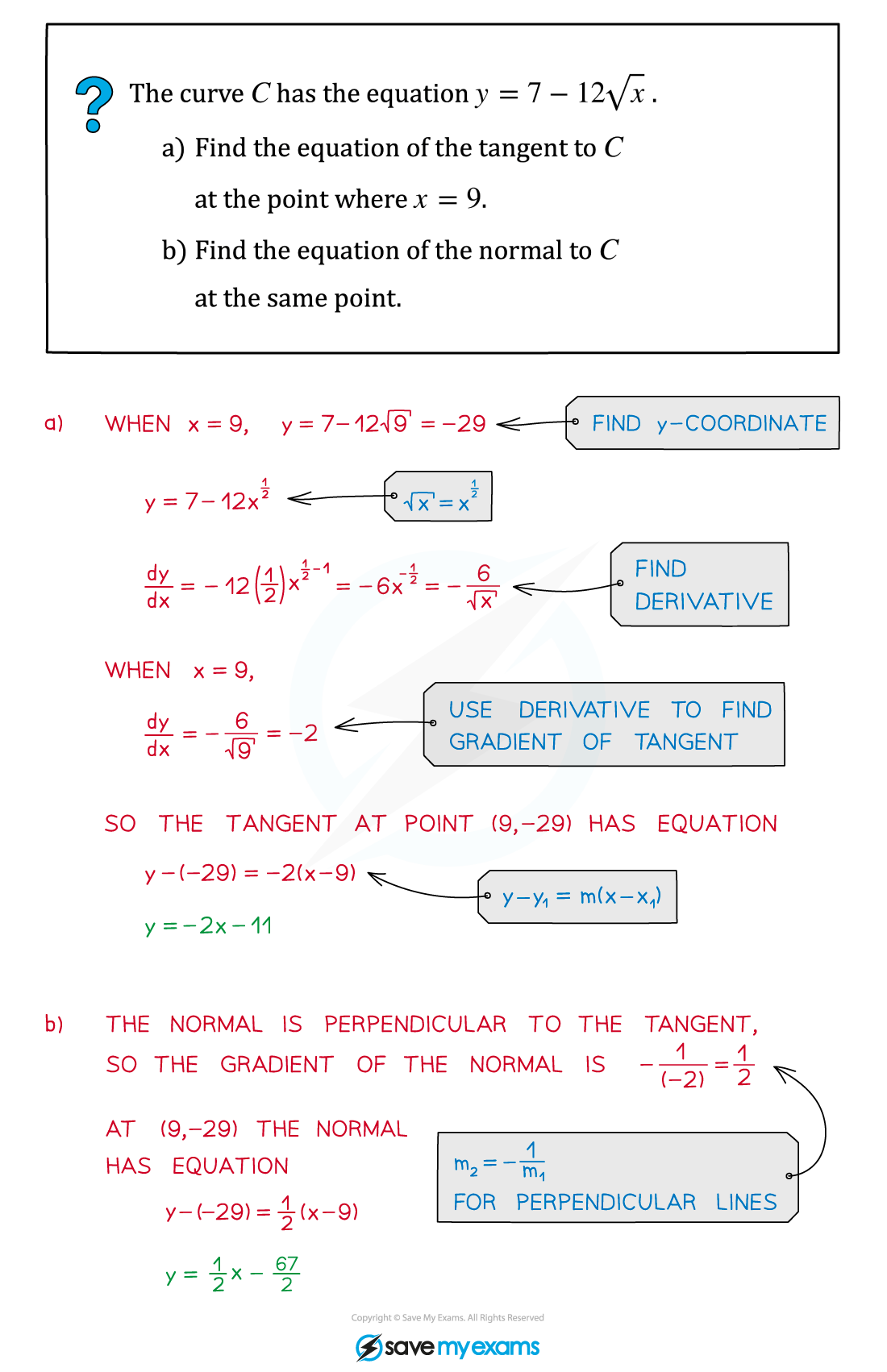# AQA A Level Maths: Pure复习笔记7.2.1 Gradients, Tangents & Normals

### Gradients, Tangents & Normals

#### Using the derivative to find the gradient of a curve

• To find the gradient of a curve y= f(x) at any point on the curve, substitute the x‑coordinate of the point into the derivative f'(x)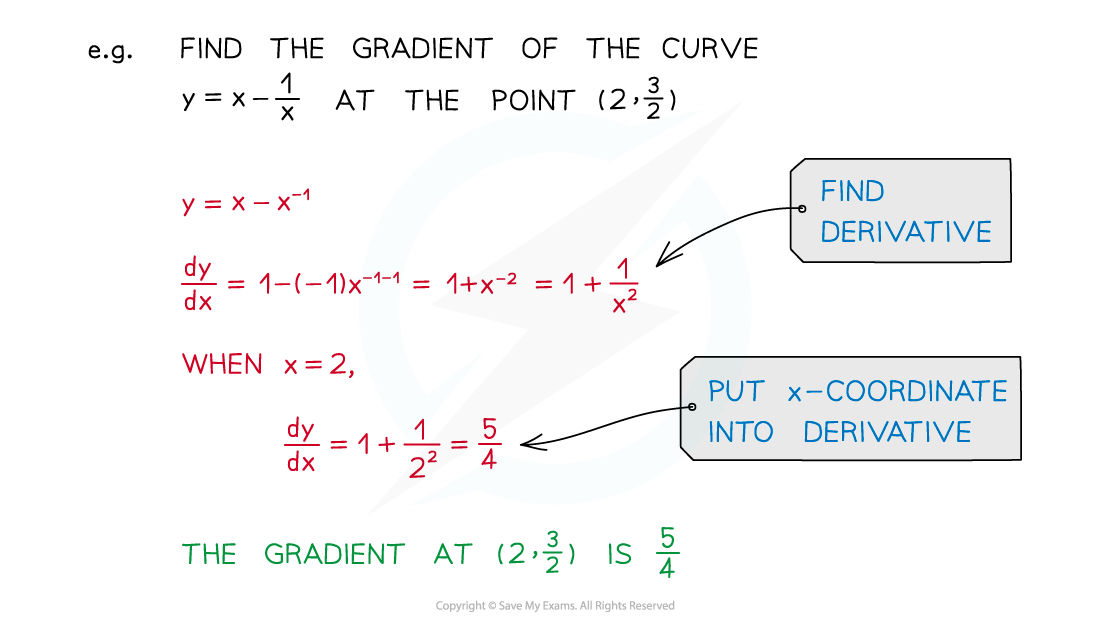#### Using the derivative to find a tangent

• At any point on a curve, the tangent is the line that goes through the point and has the same gradient as the curve at that point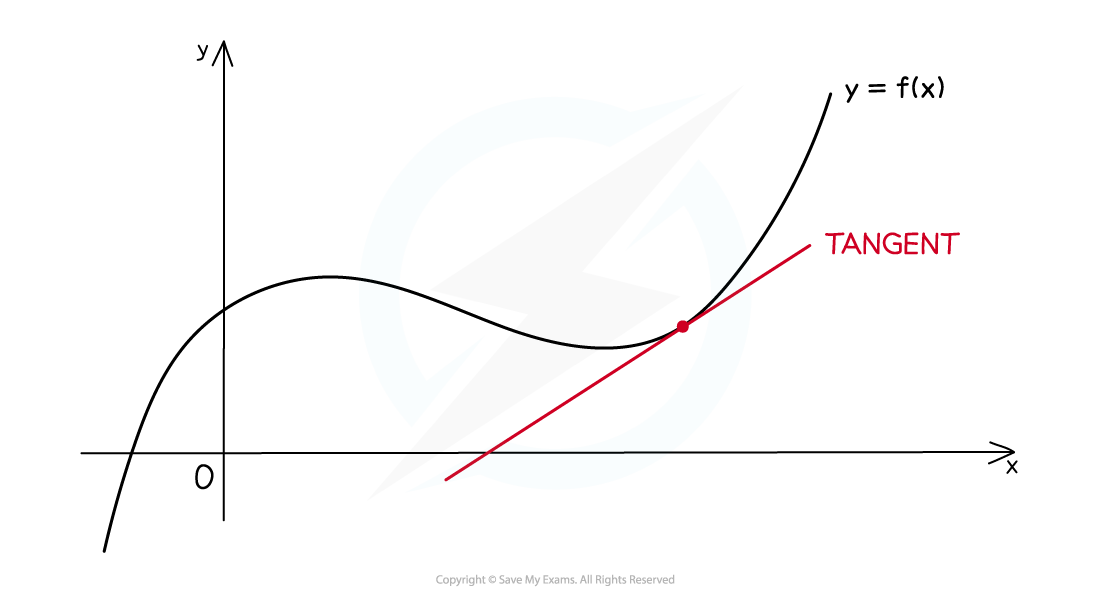####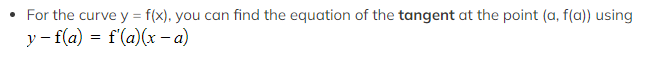Using the derivative to find a normal

• At any point on a curve, the normal is the line that goes through the point and is perpendicular to the tangent at that point####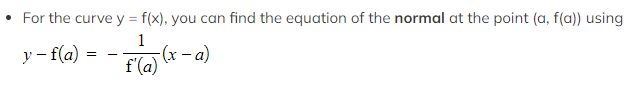Exam Tip

• The formulae above are not in the Edexcel exam formulae booklet, but if you understand what tangents and normals are, then the formulae follow from the equation of a straight line combined with parallel and perpendicular gradients (see Worked Example below).

#### Worked Example